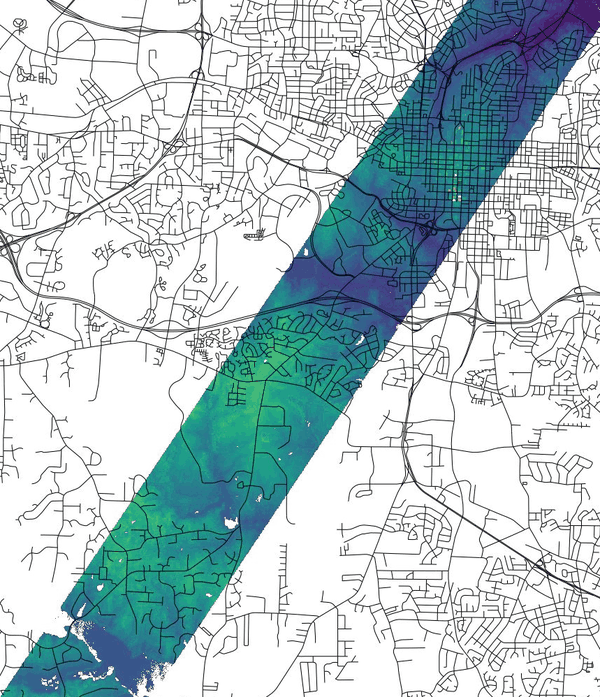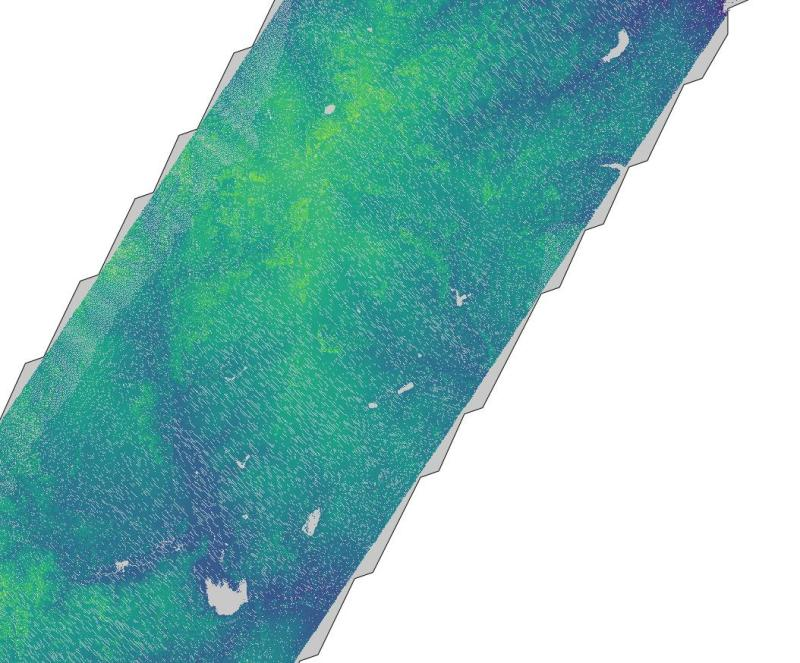## NAME

r.in.pdal - Creates a raster map from LAS LiDAR points using univariate statistics and r.in.xyz.

## KEYWORDS

raster, import, LIDAR, statistics, conversion

## SYNOPSIS

r.in.pdal
r.in.pdal --help
r.in.pdal [-sg] input=name output=name [resolution=float] [raster_reference=string] [raster_file=string] [method=string] [zrange=min,max] [zscale=float] [type=string] [percent=integer] [pth=integer] [trim=float] [footprint=string] [pdal_cmd=string] [--overwrite] [--help] [--verbose] [--quiet] [--ui]

### Flags:

-s
Scan data file for extent then exit
-g
In scan mode, print using shell script style
--overwrite
Allow output files to overwrite existing files
--help
Print usage summary
--verbose
Verbose module output
--quiet
Quiet module output
--ui
Force launching GUI dialog

### Parameters:

input=name [required]
LAS input file
output=name [required]
Name for output raster map
resolution=float
2D grid resolution (north-south and east-west)
Default: 1.0
raster_reference=string
Raster map to be used as pixel geometry reference
Raster map to align to, e.g. an orthophoto of the same region
raster_file=string
External raster map to be used as pixel geometry reference
External raster map to align to, e.g. an orthophoto of the same region
method=string
Statistic to use for raster values
Options: n, min, max, range, sum, mean, stddev, variance, coeff_var, median, percentile, skewness, trimmean
Default: mean
n: Number of points in cell
min: Minimum value of point values in cell
max: Maximum value of point values in cell
range: Range of point values in cell
sum: Sum of point values in cell
mean: Mean (average) value of point values in cell
stddev: Standard deviation of point values in cell
variance: Variance of point values in cell
coeff_var: Coefficient of variance of point values in cell
median: Median value of point values in cell
percentile: Pth (nth) percentile of point values in cell
skewness: Skewness of point values in cell
zrange=min,max
Filter range for z data (min,max)
zscale=float
Scale to apply to z data
Default: 1.0
type=string
Type of raster map to be created / Storage type for resultant raster map
Options: CELL, FCELL, DCELL
Default: FCELL
CELL: Integer
FCELL: Single precision floating point
DCELL: Double precision floating point
percent=integer
Percent of map to keep in memory
Options: 1-100
Default: 100
pth=integer
Pth percentile of the values
Options: 1-100
trim=float
Discard <trim> percent of the smallest and <trim> percent of the largest observations
Options: 1-50
footprint=string
Footprint of the data as vector map
pdal_cmd=string
Command for PDAL (e.g. if PDAL runs only in a docker)
Default: pdal

## DESCRIPTION

r.in.pdal creates a raster map from LAS LiDAR points using the Point Data Abstraction Library (PDAL) by binning the points into a raster map using univariate statistics. The user may choose from a variety of statistical methods which will be used for binning when creating the new raster map.

The computational region of a LiDAR point file can be determined by scanning the file using the -s flag.

Optionally r.in.pdal creates an estimated vector footprint area map of the LAS file when using the footprint parameter (the footprint is generated by PDAL).

Since a new raster map is created during the binning, the binning of points depends on the computational region settings (extent and resolution) which is by default set to the extent of the LiDAR input file (see more about binning below). The resulting raster resolution can be specified with the parameter resolution.

r.in.pdal is designed for processing massive point cloud datasets, for example raw LiDAR or sidescan sonar swath data. It has been tested with large datasets.

For details concerning raster binning see the manual page of r.in.lidar.

## NOTES

r.in.pdal uses g.region to set the extent and resolution of the resulting raster and internally r.in.xyz to import the LiDAR points.

## Import LAS file using PDAL

Import of a LAS file using PDAL into a mapset in the sample NC SPM location. The sample LAS file is available here.
```# check metadata
pdal info --summary lidar_raleigh_nc_spm_height_feet.las

# scan extent and exit
r.in.pdal input=lidar_raleigh_nc_spm_height_feet.las output=lidar_raleigh -s

# scan extent (g.region style) and exit
r.in.pdal input=lidar_raleigh_nc_spm_height_feet.las output=lidar_raleigh -s -g
# n=228500 s=215000.01 w=633370.82 e=645000 t=558.87 b=88.5

# set computation region to this extent
g.region n=228500 s=215000.01 w=633370.82 e=645000 -p

# import while aligning pixel geometry to existing "elevation" 10m res. raster map
# specifying EPSG manually because SRS information is missing in this LAS file
r.in.pdal input=lidar_raleigh_nc_spm_height_feet.las raster_reference=elevation \
resolution=10 output=lidar_raleigh method=mean proj_in=EPSG:3358

# optionally: footprint=lidar_raleigh_footprint

# visualize
d.mon wx0
g.list vector
d.rast lidar_raleigh
d.vect streets_wake

# analyse differences between DEM and rasterized point cloud
# LAS files come with height in US feet units
r.mapcalc "diff = elevation - lidar_raleigh * 0.3048006096012192"
r.univar -e diff
```Figure: View showing the difference of the elevation map and LiDAR point data binned to raster map with r.in.pdalFigure: LiDAR cloud footprint

## Import LAZ file using PDAL docker

Import of a LAZ file using PDAL docker into a mapset in the sample NC SPM location. The sample LAZ file is available here.
```# pulling official PDAL docker image
docker pull pdal/pdal

# using PDAL docker as command with mounted data volume
# (caution: the LAZ file has to be stored in the mounted folder! (here: \$(pwd)))
export pdal_docker="docker run --rm -v \$(pwd):/data -t pdal/pdal pdal"

# scan extent and exit
r.in.pdal input=/data/simple.laz output=lidar -s pdal_cmd="\$pdal_docker"

# scan extent (g.region style) and exit
r.in.pdal input=/data/simple.laz output=lidar -s -g pdal_cmd="\$pdal_docker"
# n=853535.43 s=848899.7 w=635619.85 e=638982.55 t=586.38 b=406.59

# set computation region to this extent
g.region n=853535.43 s=848899.7 w=635619.85 e=638982.55 -p

# import data
r.in.pdal input=/data/simple.laz output=lidar_perc95 method=percentile pth=95 pdal_cmd="\$pdal_docker"

r.univar lidar_perc95
```

r.in.xyz, r.in.lidar, v.in.lidar

## AUTHORS

Anika Bettge, mundialis GmbH & Co. KG
Vaclav Petras, NCSU GeoForAll Lab (projection, computational region)

Documentation: Markus Neteler, mundialis GmbH & Co. KG

Last changed: \$Date\$

## SOURCE CODE

Available at: r.in.pdal source code (history)

© 2003-2019 GRASS Development Team, GRASS GIS 7.8.2dev Reference Manual